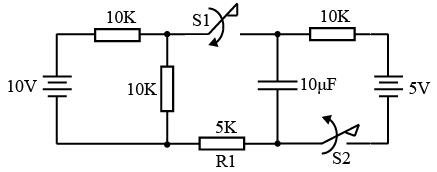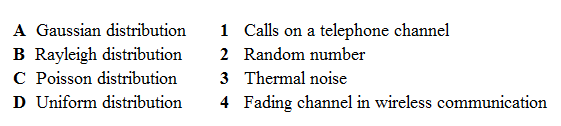# ISRO Scientist or Engineer Electronics 2014

Instructions

For the following questions answer them individually

Question 1

# In the circuit shown below, switch S1 and S2 are in open and close position respectively for long time. At $$t = t_o$$, switch S1 is closed and switch S2 is opened. What would be the current through R1 immediately after the transition of switches?Question 2

# A digital board has a unipolar square clock of 250MHz. If the clock on the board at all places should have all the harmonic components which have more than 10% of DC value, the board has to be designed forat leastQuestion 3

# A switch is connected in between a 12V battery and an uncharged capacitor and a 1 KΩ resistor. At the time instant when the switch is closed, the voltage across the capacitor is:Question 4

# Which type of timing violation will occur, If a digital IC is operated at clock frequency which is higher than its specified maximum clock frequency?Question 5

# If the maximum effective aperture of a radiating small loop of constant current is $$\left(\frac{3}{32 \pi}\right) m^2$$, the wavelength of the radiated electromagnetic wave is:Question 6

# A radar system uses magnetron as high power RF source. Its transmitter emits 300kW RF power at 10% duty factor. If the efficiency of radar transmitter during pulse is 60% and the power required during pulse off period is 1 kW..The average power dissipation in radar is,Question 7

# Match the following:Question 8

# In a fiber-optic cable, which phenomenonoccursfor signal to propagate along the inner core:Question 9

# Which of the following computation will take minimum number of clock cycles, if it is implemented on 8051 micro-controller? Following is variable declaration in c code:unsigned int a, b;Question 10

# Using a quarter wave transformer of characteristic impedance 1 Ω, a load impedance of 100 Ω will be transformed to an input impedance of value:OR